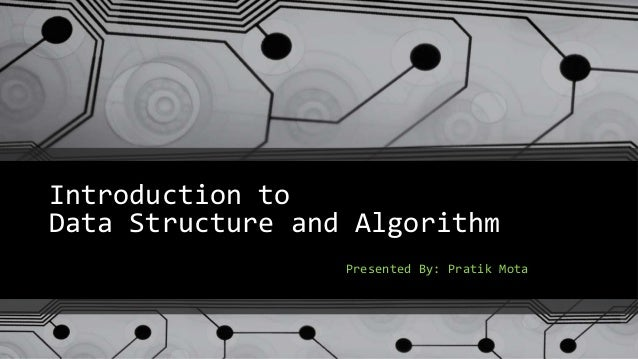Successfully reported this slideshow.Upcoming SlideShare
×

# Introduction to datastructure and algorithm

2,162 views

Published on

Introduction to data structure and algorithm

-Basics of Data Structure and Algorithm
-Practical Examples of where Data Structure Algorithms is used
-Asymptotic Notations [ O(n), o(n), θ(n), Ω(n), ω(n) ]
-Calculation of Time and Space Complexity
-GNU gprof basic

Published in: Software, Technology
• Full Name
Comment goes here.

Are you sure you want to Yes No• Be the first to comment

### Introduction to datastructure and algorithm

1. 1. Introduction to Data Structure and Algorithm Presented By: Pratik Mota
2. 2. Objectives  Basics of Data Structure and Algorithm  Practical Examples of where Data Structure Algorithms is used  Asymptotic Notations [ O(n), o(n), θ(n), Ω(n), ω(n) ]  Time and Space Complexity  GNU gprof basic
3. 3. Basics of Data structure and Algorithm  Data structure is a particular way of storing and organizing data in a computer so that it can be used efficiently.  Some Well-know Data structure 1) Array 2) List [Singly and Doubly Linked List] 3) Tree [ Binary,AVL, Red Black..etc ] 4) B+ Tree 5) Heap [ Max and Min Heap ] 6) Hashing 7) Graph [ BFS, DFS,..etc ]
4. 4. Basics of Data structure and Algorithm Algorithm is a step-by-step procedure for calculations OR you can tell it is sequence of program instructions designed to compute a particular result.  Examples:- Sorting, Searching, Shortest Path, Dynamic Programming, Numerical Algorithms etc.. Sort INPUT sequence of numbers a1, a2, a3,….,an b1,b2,b3,….,bn OUTPUT a permutation of the sequence of numbers 2 5 4 10 7 2 4 5 7 10 Sorting Algorithm
5. 5. Use of Data Structure and Algorithm
6. 6. Practical Examples (Cont.)
7. 7. Practical Examples (Cont.)
8. 8. Asymptotic Notations 1n 2n 3n 4n 5n 6n Runningtime 1 2 3 4 5 6 7 8 9 10 11 12 ….. best-case Ω(n) average-case θ(n) worst-case O(n) Input instance size o(n) ω(n)
9. 9. Basics of Complexity O(1) < O(log n ) < O(n) < O(n log n ) < O(n^2) < ….. < O(n^k) < O(2^n) O( n^2 + n + 1 ) => O(n^2)  MAX ( f(n), g(n) )  O(N + a)^b  O(N^b) Time Complexity :- Amount of time taken by an algorithm to run. Space Complexity:- Extra/Temporary Space use by algorithm .
10. 10. Time and Space Complexity O(n) O(n^2) O( log n )
11. 11. GNU gprof  Gprof is a profiling program which collects performance statistics of our programs.  For Performance analysis -pg option needed for GCC / g++ Compiler. Ex:- g++ -pg gprof_test.cpp -o gprof_test  After running ./gprof_test , It generates gmon.out file.  gmon.out file contain Performance analysis statistics, which can be analyze using gprof tool. Ex:- gprof gprof_test gmon.out > analysis.txt  It provides mainly two type of Performance Analysis 1) Flat Profile [ Total amount of time your program spent executing each function ] 2) Call graph [ How much time was spent in each function and its children ]